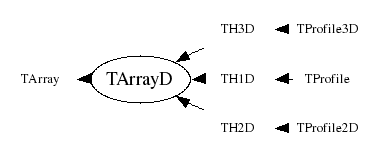library: libCore#include "TArrayD.h"

# TArrayD

class description - header file - source file

## class TArrayD: public TArray### Function Members (Methods)

Display options:
Show inherited
Show non-public
public:
 TArrayD() TArrayD(Int_t n) TArrayD(const TArrayD& array) TArrayD(Int_t n, const Double_t* array) virtual ~TArrayD() void AddAt(Double_t c, Int_t i) void Adopt(Int_t n, Double_t* array) Double_t At(Int_t i) const static TClass* Class() void Copy(TArrayD& array) const const Double_t* GetArray() const Double_t* GetArray() Int_t TArray::GetSize() const Stat_t GetSum() const virtual TClass* IsA() const TArrayD& operator=(const TArrayD& rhs) Double_t& operator[](Int_t i) Double_t operator[](Int_t i) const static TArray* TArray::ReadArray(TBuffer& b, const TClass* clReq) void Reset() void Reset(Double_t val) virtual void Set(Int_t n) void Set(Int_t n, const Double_t* array) virtual void ShowMembers(TMemberInspector& insp, char* parent) virtual void Streamer(TBuffer& b) void StreamerNVirtual(TBuffer& b) static void TArray::WriteArray(TBuffer& b, const TArray* a)
protected:
 Bool_t TArray::BoundsOk(const char* where, Int_t at) const Bool_t TArray::OutOfBoundsError(const char* where, Int_t i) const

### Data Members

public:
 Double_t* fArray [fN] Array of fN doubles Int_t TArray::fN Number of array elements

## Class Description

```
TArrayD

Array of doubles (64 bits per element).

```
TArrayD()
``` Default TArrayD ctor.
```
TArrayD(Int_t n)
``` Create TArrayD object and set array size to n doubles.
```
TArrayD(Int_t n, const Double_t *array)
``` Create TArrayD object and initialize it with values of array.
```
TArrayD(const TArrayD &array)
``` Copy constructor.
```
TArrayD & operator=(const TArrayD &rhs)
``` TArrayD assignment operator.
```
~TArrayD()
``` Delete TArrayD object.
```
``` Adopt array arr into TArrayD, i.e. don't copy arr but use it directly
in TArrayD. User may not delete arr, TArrayD dtor will do it.
```
``` Add double c at position i. Check for out of bounds.
```
void Set(Int_t n)
``` Set size of this array to n doubles.
A new array is created, the old contents copied to the new array,
then the old array is deleted.
This function should not be called if the array was declared via Adopt.
```
void Set(Int_t n, const Double_t *array)
``` Set size of this array to n doubles and set the contents
This function should not be called if the array was declared via Adopt.
```
void Streamer(TBuffer &b)
``` Stream a TArrayD object.
```

void Copy(TArrayD &array)
`{array.Set(fN,fArray);}`
const Double_t * GetArray()
`{ return fArray; }`

`{ return fArray; }`

`{Stat_t sum=0; for (Int_t i=0;i<fN;i++) sum+=fArray[i]; return sum;}`
void Reset()
`{memset(fArray, 0, fN*sizeof(Double_t));}`
void Reset(Double_t val)
`{for (Int_t i=0;i<fN;i++) fArray[i] = val;}`

Author: Rene Brun 06/03/95
Last update: root/cont:\$Name: \$:\$Id: TArrayD.cxx,v 1.14 2005/11/16 20:07:50 pcanal Exp \$